Courses
Courses for Kids
Free study material
Offline Centres
More

# Chord of CircleLast updated date: 26th Nov 2023
Total views: 190.5k
Views today: 5.90k## Chord in Maths: Introduction

The line segment connecting any two points on a circle's circumference is known as the chord of a circle. A line segment which passes through the center of the circle is the longest chord of the circle which is known as diameter. In this article, we will learn about the chord of circle, the formula for a chord of circle, and its theorems. We will learn different words like ‘circumference ’, ‘diameter’, etc

## What is the Chord of a Circle?

The line segment connecting any two points on a circle's circumference is known as the chord of a circle. So, the chord is one whose endpoints lie on the circumference. We can say that diameter is also the chord which is the longest and also passes through the center of the circle. The chord is one of the various line segments that may be made in a circle whose ends are on the circumference. A chord that runs through the center of the circle is also known as the diameter.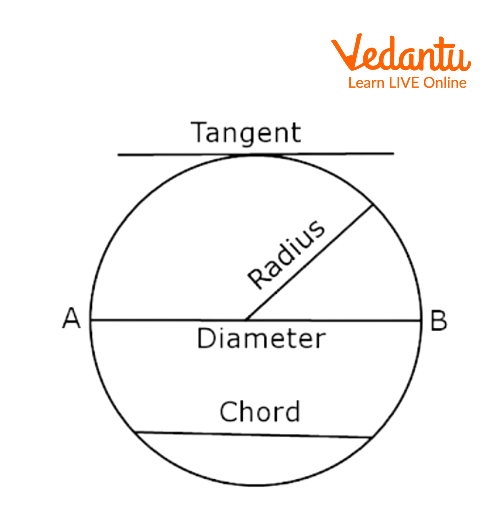Keywords of a Circle

## Properties of the Chord of a Circle

• When a perpendicular is drawn to the chord from the center of the circle then it bisects the chord.

• Chords of the circle which are equidistant from the center of the circle are equal.

• When there are three collinear points then they pass through one and only one circle.

• When we draw the chord of the circle, it divides the circle into two segments i.e. major segment and minor segment.

• Well a secant is the one when the chord is extended infinity on both the sides.

## Formula for the Chord Length of a Circle

Well there are two common formulas which are used to find the length of the chord. They are:

• Using the Perpendicular Distance from the Center: The Chord Length is $2\sqrt{{{r}^{2}}-{{d}^{2}}}$, where ‘r’ is the radius of the circle and ‘d’ is the perpendicular distance from the chord to the center of the circle.Perpendicular on the Chord of the Circle

• Using Trigonometry: The Chord Length is $2r\sin \left( {\frac{\theta }{2}} \right)$ , where ‘r’ is the circle's radius and ‘$\theta$’ is the angle subtended at the center by the chord.

## Theorem of Unequal Chords

Statement: In a circle with two unequal chords, the larger chord will be closer to the center than the smaller chord.

Given: We have drawn chords CD and AB.

To prove that: The larger chord will be closer to the center.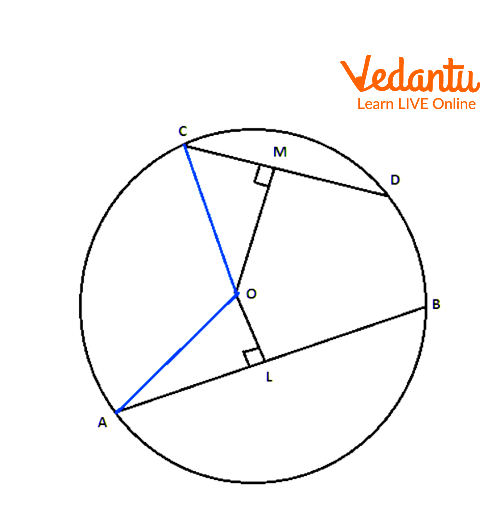Two Unequal Chords

Proof:

We can see that, chord AB is near to the center and chord CD is away from the center. The length of the chord increases as it moves near to the center. Here, we can see that length of the chord AB is longer than the chord CD. This means, that we move away from the center the length of the chord decreases. From this, we can say that diameter is the longest chord.

Hence proved.

## Theorem of Equal Chords, Equal Angles

Statement: At the circle's center, chords with identical lengths subtend equal angles.

Proof: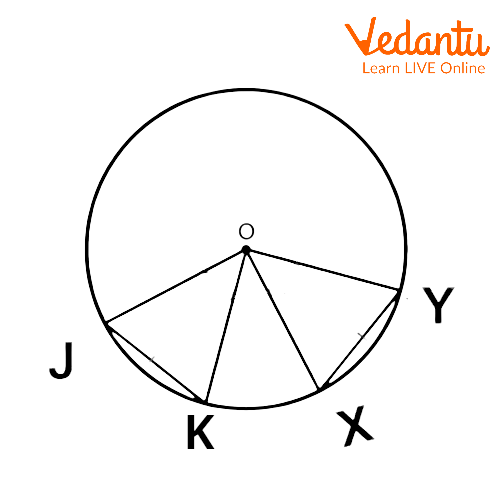Equal chords

Given that $O$ is a center.

$JK$ and $XY$ are equal chords of the circle.

That is, $JK=XY$.

In $\Delta \text{ }JOK$ and $\Delta \text{ }XOY$,

Radii: $OJ=OK=OX=OY$

According to the SSS rule, two triangles are congruent if all three sides of one triangle are equal to the corresponding three sides of the second triangle.

So, $\Delta \text{ }JOK\cong \Delta \text{ }XOY$

By CPCT rule

$\angle JOK=\angle XOY$

Hence proved.

## Converse Theorem of Equal Chords

Statement: The length of the chords is equal if and only if the angles of a circle's chords subtend are the same size.

Proof: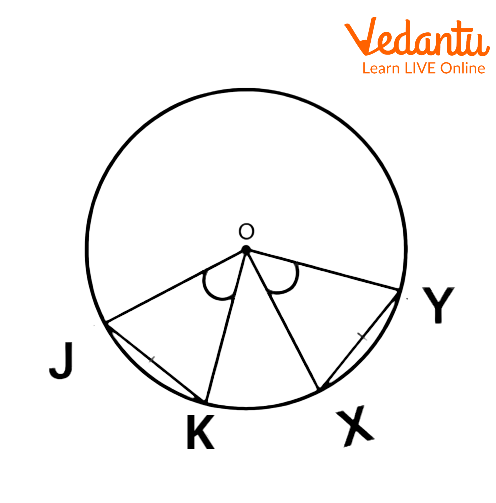Converse Theorem of Equal Chords

Given that $O$ is the center of the circle.

$JK$and $XY$ are the chords that subtend equal angles at center $O$.

So, $\angle JOK=\angle XOY$

In $\Delta \text{ }JOK$ and $\Delta \text{ }XOY$,

Radii: $OJ=OK=OX=OY$

According to the SAS rule, two triangles are said to be congruent if all two sides and one angle of one triangle are equal to the corresponding two sides and one angle of the second triangle.

So, $\Delta \text{ }JOK=\Delta \text{ }XOY$

By the CPCT rule,

$JK=XY$

Hence proved.

## Interesting Facts

• A circle's radius intersects the chord at a 90° angle.

• Two radii join a chord's two ends to create an isosceles triangle.

• The three provided non-collinear points are intersected by one and only one circle.

## Solved Important Questions

1. We have a circle with center O and having 8 cm as the radius. Find the length of the chord if the length of the perpendicular drawn from the center is 10 cm.

Ans: Let AB be the chord of the circle with center O and let OM is perpendicular to the chord.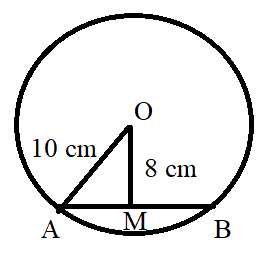Given Length of Perpendicular

As we can see, triangle OMA is a right angled triangle. So by using Pythagoras theorem, we can say that,

$\therefore O{A^2} = O{M^2} + A{M^2}$

$\Rightarrow A{M^2} = O{A^2} - O{M^2}$

$\Rightarrow A{M^2} = {10^2} - {8^2}$

$\Rightarrow A{M^2} = 36$

$\Rightarrow AM = 6$

Also, we know that, Am = MB and AB = AM+MB = 6 + 6 = 12 cm

Thus, the length of the chord is 12 cm.

2. If a circle is formed when two points connected by a line segment subtend equal angles at two additional points located on the same side of the line as the line containing the line segment (i.e., they are concyclic). Is this statement true?

Ans: P, Q, R, and S are four points

PQ subtends equal angles at R and S

That is, $\angle PR\text{Q=}\angle P\text{SQ}$

We have to prove that P, Q, R, and S are cyclic

Since P, Q, and R, are non-collinear.

Consider, a circle passes through three collinear points

Let us draw a circle with the center at $O$.Points P, Q, R are Non-collinear

Let us assume it does not lie in the circle.

Angles within a segment are now equal.

$\angle PR\text{Q=}\angle P\text{{S}'Q}$—(1)

Given that,

$\angle PR\text{Q=}\angle P\text{SQ}$—(2)

From (1) and (2),

$\angle P\text{{S}'Q}=\angle P\text{SQ}$

In $\Delta \text{ QS{S}'}$,

By exterior angle property

$\angle P\text{{S}'Q}=\angle QS\text{{S}'+}\angle \text{{S}'}QS$

From (1),

$\angle P\text{SQ}=\angle P\text{SQ+{S}'}QS$

$\angle P\text{SQ-}\angle P\text{SQ={S}'}QS$

$\therefore \text{{S}'}QS=0$

So, $\text{{S}'}$ and $S$ are coincide

Hence, our assumption was wrong.

Point $S$ lies on circle

Thus, P, Q, R and S are concyclic.

Hence this statement is true.

3. Find the angle subtended by this chord at a point in the major segment if given that the chord of the circle is equal to its radius.

Ans: Let point O be the center of the circle and AB be the chord of the circle.

Also, we can see that OA and OB are the radii of the circle.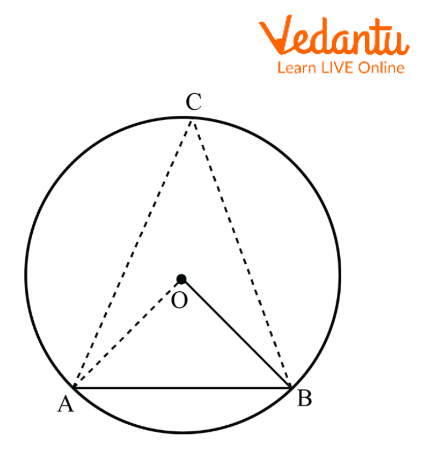Chord is Equal to the Radius of Circle

Given that the chord of the circle is equal to the radius. This means AB = OA =OB.

Also, we can say that triangle AOB is an equilateral triangle.

$\angle AOB = \angle OBA = \angle OAB = {60^ \circ }$

As we know, the angle subtended by the arc at the center of the circle is twice the angle subtended by it at any other point in the remaining part of the circle.

$\therefore \angle AOB = 2\angle ACB$

$\Rightarrow \angle ACB = \frac{1}{2}\angle AOB$

$\Rightarrow \angle ACB = \frac{1}{2}{60^ \circ } = {30^ \circ }$

Hence, the angle subtended by the given chord at a point in the major segment is ${30^ \circ }$.

## Conclusion

In this article, we learned the chord meaning, chord Properties of a circle, the formula for the chord of a circle, its theorems and the chord of a circle example. The line segment connecting any two points on a circle's circumference is known as the chord of a circle. Note that the diameter (also known as chord of the circle) is the longest chord of a circle which passes through the center of the circle.

## Practice Questions

1. Chord, meaning in Latin, is

A. Bowstring

B. String

C. Line

D. Group

2. When the chord's perpendicular distance from the circle's center is $6\text{ }cm$ and the circle's radius is $9\text{ }cm$, determine the chord's length.

A. $13.014\text{ }cm$

B. $13.316\text{ }cm$

C. $13.4164\text{ }cm$

D. $13.2164\text{ }cm$

3. Equation of chord

A. $2\sqrt{{{r}^{2}}-{{d}^{2}}}$

B. $2\sqrt{r+d}$

C. $2\sqrt{{{r}^{2}}+{{d}^{2}}}$

D. $2\sqrt{r-d}$

1) A

2) C

3) A

## FAQs on Chord of Circle

1. What is the relationship between the chord of a circle and a perpendicular to it from the center?

As we know that when a perpendicular is drawn from the center of the circle to the chord, then it bisects the chord. Thus, a line drawn through the center of a circle bisects a chord is always perpendicular to the chord.

2. Is chord same as the radius of the circle?

A line segment connecting two points along a circle's circumference is known as the chord of a circle. The circle's circumference, which passes through its center, is the circle's longest chord. So diameter is also known as the chord of the circle which is the longest chord of the circle. And radius of a circle is the one which connects the center and any one point on the circumference of the circle. Hence, we can say that the radius cannot be called as a chord.

3. What are the different types of Chords?

We know that a chord is a straight line which joins two points on the perimeter of a shape, especially a circle. There are four main different types of Chords and they are:

• Major Chords

• Minor Chords

• Diminished Chords

• Augmented Chords